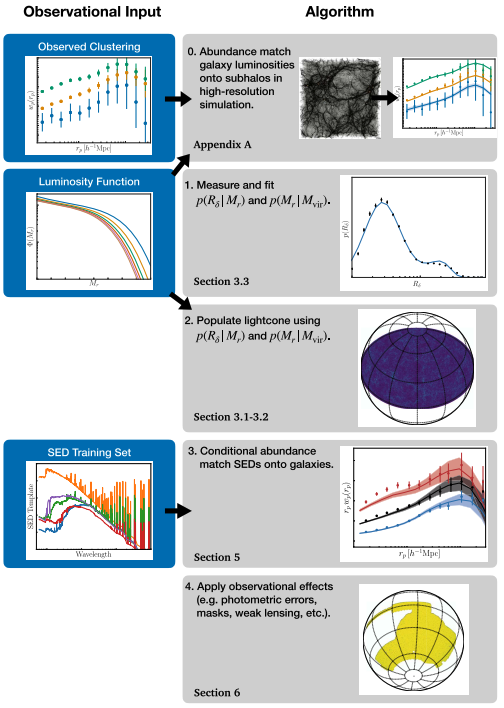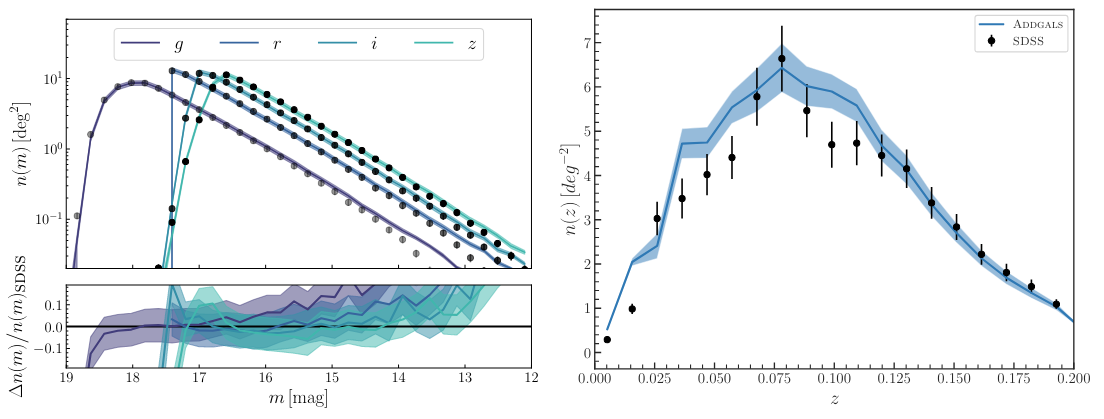# Adding Gal-ax-ies, It’s Easy as One-Two-Three

Authors: Risa H. Wechsler, Joseph DeRose, Michael T. Busha, et al.

First Author’s Institution: Department of Physics, Stanford University, Stanford, CA; Kavli Institute for Particle Astrophysics & Cosmology, Stanford, CA; SLAC National Accelerator Laboratory, Menlo Park, CA

Status:  Published in ApJ [open access]

In recent years, the fields of cosmology and extragalactic astronomy have experienced an explosion of scientific discoveries, driven by advancements in numerical simulations of cosmology and galaxy formation in tandem with the proliferation of increasingly large volumes of observational data. In our current understanding of the universe’s evolution, galaxy formation and cosmology are intimately linked, with galaxies serving as the tracers of the large-scale matter distribution in the universe as they are fundamentally connected to their host dark matter (DM) halo. In other words, in order to study the underlying dark matter distribution in the universe and probe the fundamental physics that underlies the cosmology we observe today, we must rely on statistically significant samples of galaxy observations.

Ideally, in order to interpret such observations, one can imagine numerically simulating the entire history of the universe with all the underlying physics included – as is done in cosmological hydrodynamic simulations – and comparing the resulting galaxy populations to observed samples. However, this is a computationally expensive task and is thus not so easily scalable to large volumes (such as the size of our universe). Instead, we often utilize quicker approaches to produce synthetic catalogs of galaxies that rely on our understanding of the physical connection between galaxies and DM halos, such as halo occupation distributions (which describe how many galaxies we expect to find in a given DM halo and where we expect to find them), semi-analytic models (wherein physical processes are ‘painted’ onto a numerical simulation of gravitational structure formation), and subhalo abundance matching (where galaxies are assigned to their host halos by matching the number density of galaxies above a certain mass to the number density of DM halos above a certain mass). Crucially, these approaches all require a reasonably high resolution simulation of DM structure formation from which the galaxy population can be built up. Inspired by the utility of mock catalogs but the simultaneous lack of computationally efficient tools to build them, today’s authors present ADDGALS (Adding Density-Determined Galaxies to Lightcone Simulations), which provides a relatively computationally inexpensive approach to construct realistic, synthetic galaxy catalogs from modest-resolution simulations of DM structure.

Broadly, the ADDGALS algorithm can be summarized in the steps given in Figure 1. That is, the authors leverage a machine learning inspired approach to use data from a high resolution simulation to fit their models for the connection between galaxies and their DM halos and survey data to fit a model that connects galaxy properties to the local DM density distribution, such that both sets of models can then be applied to a lower resolution simulation to produce a mock catalog of galaxies. Put simply, given a simulation of dark matter, this algorithm helps figure out where the galaxies should be placed and how they should appear if observed in a survey.Figure 1: A flowchart summarizing the steps of the ADDGALS algorithm. The observational inputs to the model – the clustering, luminosity function, and spectral energy distributions for galaxies – are shown at the left and the corresponding steps of the algorithm are given on the right. (adapted from Figure 1 in the paper)

Therefore, the first part of the algorithm is to figure out where the galaxies need to go (steps 0-2 in Figure 1). The authors split this process into two steps. First, they assign the largest, the so-called ‘central’, galaxies to their halos by assuming a statistical relationship between the halo mass and central galaxy magnitude, the p(Mr|Mvir) in Steps 1 and 2 of Figure 1. Then, they need to populate the halos with satellite galaxies using a relationship between large-scale DM density and galaxy magnitude, the p(R|Mr, z) displayed in Figure 1. This distribution, for which they assume a form based on their simulations, gives the probability that we’d see a galaxy of a magnitude, Mr, at a redshift of z in a region of DM density R (technically this is the size of the region so is denoted R, but is associated with a fixed mass, so we can think of it as a density). The authors then calibrate these two relationships based on a high-resolution simulation. With these two calibrated probability distributions – the central brightness-halo mass and density-magnitude relations – in hand, they can take a lower resolution simulation and statistically assign galaxies to the simulated DM halos, yielding a catalog of galaxies with positions, velocities, and magnitudes.

The next step is to associate spectral energy distributions (SEDs) – the curves describing an object’s emitted energy versus wavelength – with the galaxies that have been placed in the simulation (steps 3-4 in Figure 1). This is done in a similar, statistical manner to the assignment of galaxies to halos, except this time the authors use a sample of 600,000 real galaxies from the Sloan Digital Sky Survey (SDSS) to serve as their ‘training set’ of SEDs. In order to figure out which SED goes with which synthetic galaxy, the authors find that they can use distance to the nearest massive DM halo as a good proxy for observed g-r color. At a fixed galaxy magnitude, this means that they can use abundance matching to associate colors with galaxies depending on the local DM environment. They then pick the SEDs that are associated with the color value assigned to the galaxy and use these to compute an observed magnitude in each band for these galaxies. To realistically compare these synthetic catalogs to data observed in a real survey (which will, for example, have a limiting magnitude that it can observe), the authors also introduce synthetic errors, such as noise from the galaxy’s flux and the sky.

Finally, the authors demonstrate the validity of this approach by comparing the galaxy distribution generated by ADDGALS to actual SDSS data, such as that shown in Figure 2, showing good agreement between the observed number and color of galaxies at various redshifts and magnitudes. The algorithm described in today’s paper provides a computationally inexpensive way to produce mock survey data and has already been applied to a variety of surveys and contexts, for example allowing scientists to easily test methodology that can be applied to forthcoming surveys and evaluate cosmological probes that have been applied to existing surveys.

While the work in this paper isn’t quite as easy as A-B-C or Do-Re-Mi, today’s authors have done their best to make synthetic galaxy catalog construction as easy as (following steps) 1, 2, 3 (, and 4 in Figure 1)!Figure 2: The number density as a function of magnitude (left) and redshift (right) for the synthetic catalog (colored curves) compared to SDSS data (black points). The plot at the upper left shows agreement in several different bands, and the plot at the lower left quantifies the agreement, displaying the error as a function of magnitude. (adapted from Figure 5 in the paper)

Astrobite edited by Graham Doskoch